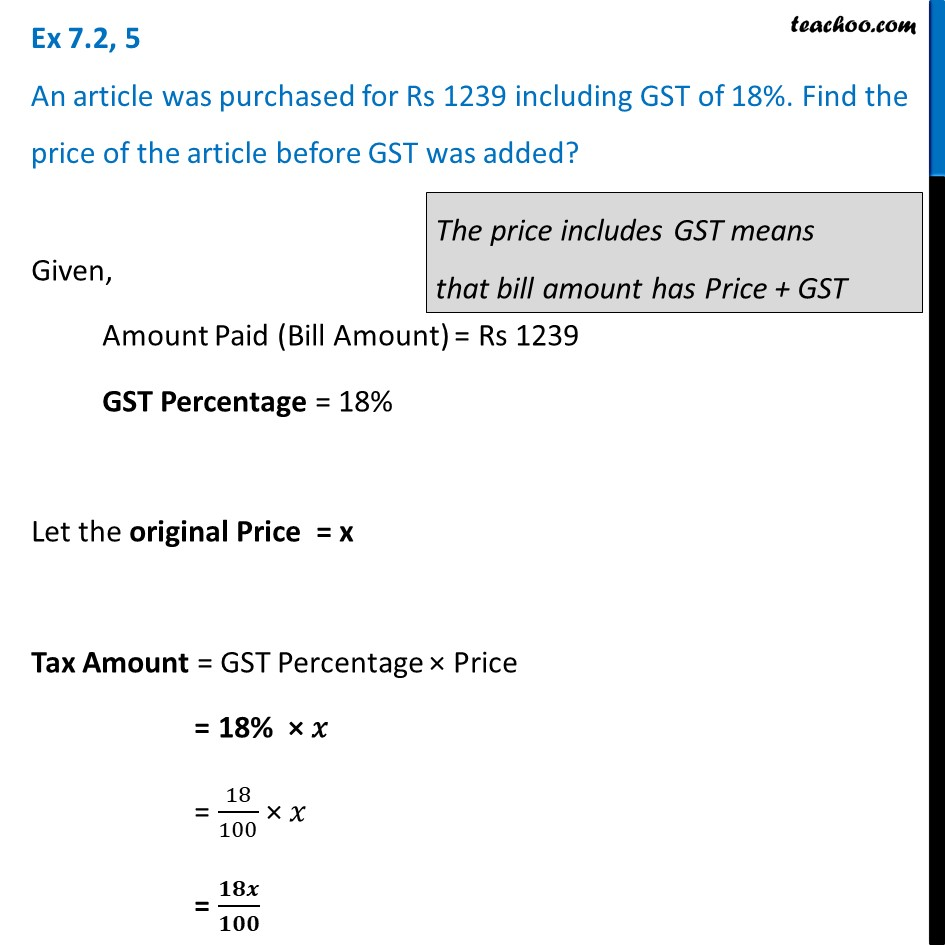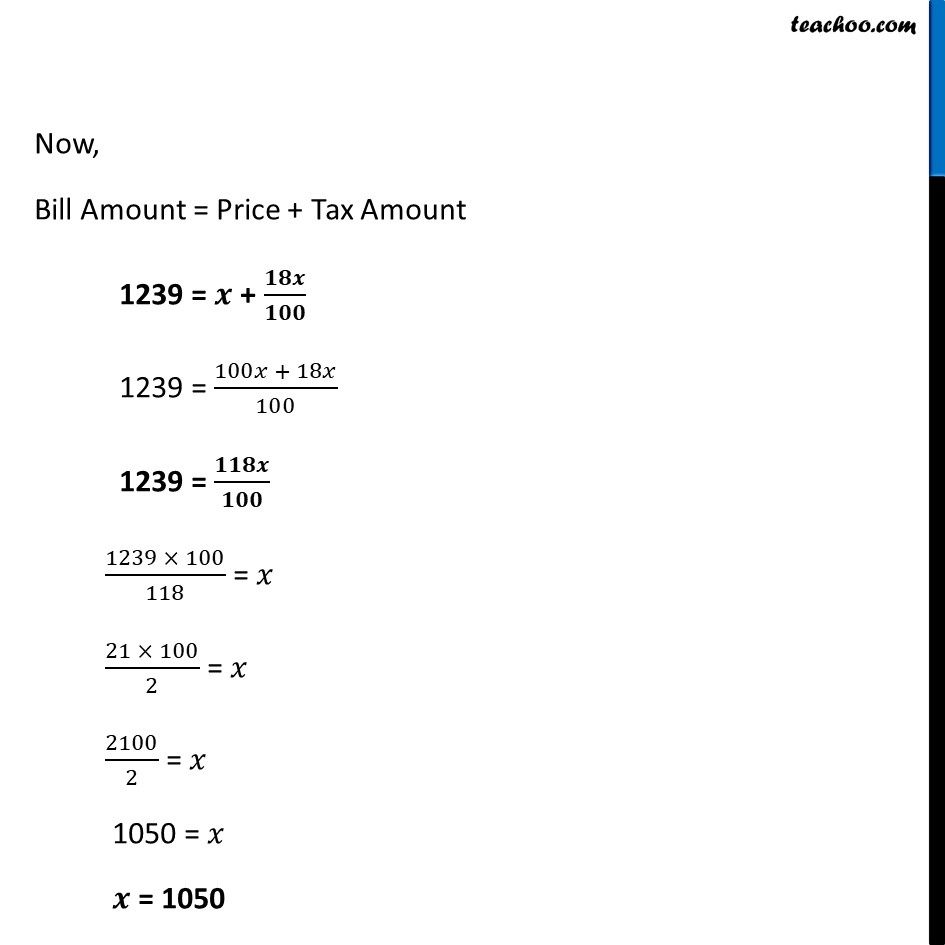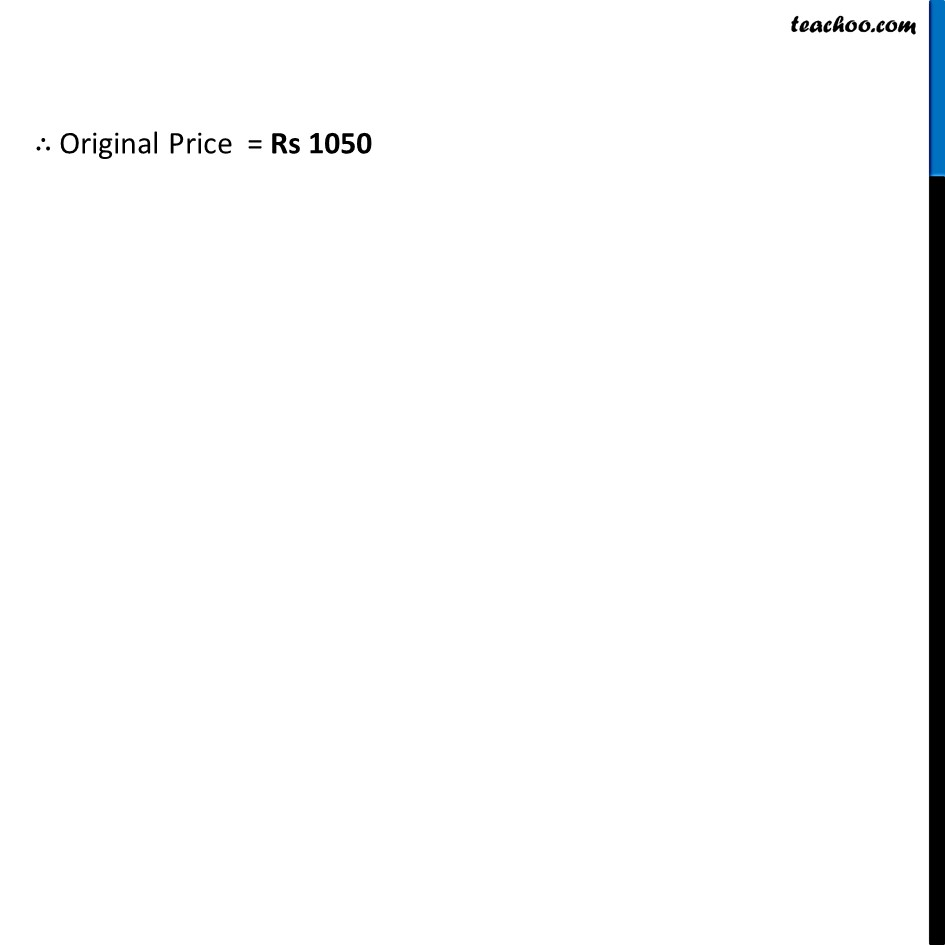Ex 7.2

Chapter 7 Class 8 Comparing Quantities
Serial order wiseLearn in your speed, with individual attention - Teachoo Maths 1-on-1 Class

### Transcript

Ex 7.2, 5 ﻿An article was purchased for Rs 1239 including GST of 18%. Find the price of the article before GST was added?Given, Amount Paid (Bill Amount) = Rs 1239 GST Percentage = 18% Let the original Price = x Tax Amount = GST Percentage × Price = 18% × 𝑥 = 18/100 × 𝑥 = 𝟏𝟖𝒙/𝟏𝟎𝟎 Now, Bill Amount = Price + Tax Amount 1239 = 𝒙 + 𝟏𝟖𝒙/𝟏𝟎𝟎 1239 = (100𝑥 + 18𝑥)/100 1239 = 𝟏𝟏𝟖𝒙/𝟏𝟎𝟎 (1239 × 100)/118 = 𝑥 (21 × 100)/2 = 𝑥 2100/2 = 𝑥 1050 = 𝑥 𝒙 = 1050 ∴ Original Price = Rs 700 ∴ Original Price = Rs 1050